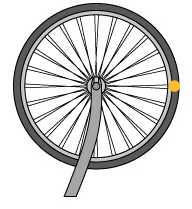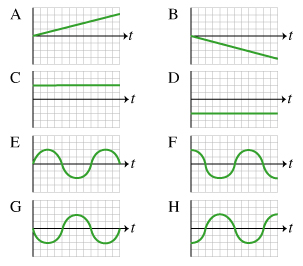# Problem: While working on her bike, Amanita turns it upside down and gives the front wheel a counterclockwise spin. It spins at approximately constant speed for a few seconds. During this portion of the motion, she records the x and y positions and velocities, as well as the angular position and angular velocity, for the point on the rim designated by the yellow-orange dot in the figure. Let the origin of the coordinate system be at the center of the wheel, the positive x-direction to the right, the positive y position up, and the positive angular position counterclockwise. The graphs (figure 2) begin when the point if at the indicated position. One graph may be the correct answer to more than one part1. Which of the graphs corresponds to x position versus time?2. Which of the graphs corresponds to angular position versus time?3. Which of the graphs corresponds to y velocity versus time?4. Which of the following graphs corresponds to angular velocity versus time?

###### FREE Expert Solution

1.

Drawing the x and y axes over the wheel, we notice that coordinate x starts with a positive value.

A sinusoidal function best describes the movement in the x-axis since it is a circular trajectory.

88% (306 ratings)###### Problem Details

While working on her bike, Amanita turns it upside down and gives the front wheel a counterclockwise spin. It spins at approximately constant speed for a few seconds. During this portion of the motion, she records the x and y positions and velocities, as well as the angular position and angular velocity, for the point on the rim designated by the yellow-orange dot in the figure.Let the origin of the coordinate system be at the center of the wheel, the positive x-direction to the right, the positive y position up, and the positive angular position counterclockwise. The graphs (figure 2) begin when the point if at the indicated position. One graph may be the correct answer to more than one part1. Which of the graphs corresponds to x position versus time?

2. Which of the graphs corresponds to angular position versus time?

3. Which of the graphs corresponds to y velocity versus time?

4. Which of the following graphs corresponds to angular velocity versus time?